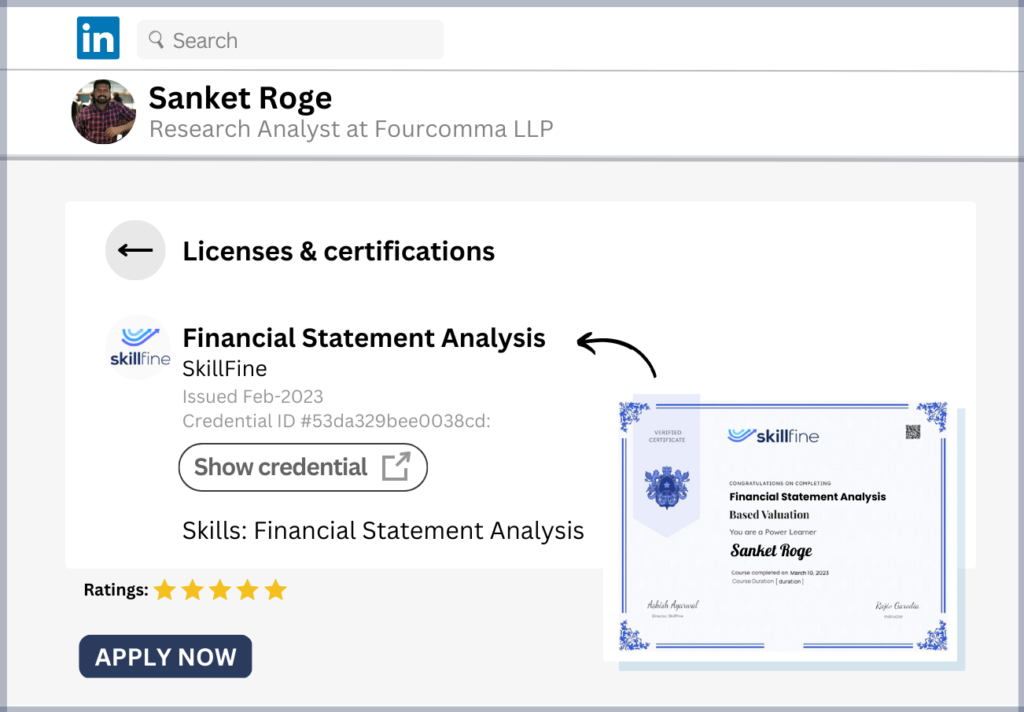# Master Text Functions in Excel to Analyze Data Like a Pro## Master Text Functions in Excel to Analyze Data Like a Pro

Excel is the most frequently used data analysis tool worldwide. As such mastering it is critical to undertake any useful analysis for work purposes. There are a number of very useful TEXT functions in Excel that can make very powerful analysis in any dataset.

A list of the most important TEXT functions in Excel are below:

### 1. LEFT

This function extractsa certain number of the leftmost characters from a string. The Syntax for this function is

=LEFT (text, num_char))

Where “text” refers to the string from which we need to extract the characters and “num_char” represents the number of characters we want to extract.

Example:

=LEFT(“Excel”,2) would give us the result as “Ex” i.e. it extracts the 2 left most characters from the string “Excel”

### 2. LEN

This function helps to know the length of a string, that is number of characters in a string. The syntax of this function is

= LEN(text)

Where “text” refers to the string whose length we want to extract.

Example:

=LEN(“Excel”) would give us the result as 5 i.e. there are 5 characters in this string.

### 3. MID

This function helps to extract a specified number of characters from the middle of a string. The syntax of this function is

= MID(text, start_char, num_chars)

Where “text” is the string from which we want to extract data, “start_char” is the starting position of the character from which data should be extracted and “num_char” is the number of characters that needs to be extracted.

Example:

=MID (“Excel”,2,3) would give us the result as “xce” i.e. it would start from the second position (included) and give us 3 characters from there.

### 4. FIND

This function helps to know the position of certain characters in a particular string. The syntax of this function is

=FIND(find_text, within_text,[start_num])

Where “find_text” is the string we want to search, “within_text” is the parent string within which we want to conduct the search and “start_num” is an optional argument to specify the starting point of the search. If nothing is specified it takes 1 as the default value.

Example:

=FIND(“e”,”Excel”,1) would give us the result as 4.

Note this function is case sensitive. So in this it will return the position of small-case “e” and not capital “E”

### 5. PROPER

This function is usedto capitalize each word in the string that is, it converts the starting alphabet of each word in a string to capital case. The syntax of the function is

=PROPER(text)

where “text” is the string that we want to convert into proper case.

Example:

=PROPER(“Excel is a data analysis tool”) would give us the result as “Excel Is A Data Analysis Tool”. Notice how the starting alphabet of each word has been capitalized in this function.

### 6. REPT

This function is used when we want a certain text to be repeated certain number of times. The syntax of this function is

=REPT(Text, number_times)

Where “text” is a string we want to repeat and “number_times” is the number of repetitions we want to have.

Example:

=REPT(“Excel”,4) will give us the result as “ExcelExcelExcelExcel”. Notice it exactly repeats the text 4 times back to back with no spaces between the repetitions.

### 7. TRIM

This function is used to remove the unnecessary spaces in between words from a particular string. The syntax for this formula is:

=TRIM(text)

Where “text” is the string from where we need to removed the unwanted spaces.

Example:

=TRIM(“   Excel    is a Data analysis     tool”) will give us the result as “Excel is a Data analysis tool”. Notice all unwanted blank spaces between words is removed and the standard 1 space between words is kept. Any space at the beginning of the first word is completely removed.

### 8. UPPER

This function convertsall the text into Upper case from lower case. The syntax of this function is

=UPPER(Text)

Where text is the string that we want to convert into upper case.

Example:

=UPPER(“Excel is a Data analysis tool”) will give us the result as “EXCEL IS A DATA ANALYSIS TOOL”. Notice all the words are completely converted into upper case.

### 9. RIGHT

This function extractsa certain number of the rightmost characters from a string. The Syntax for this function is

=RIGHT (text, num_char))

Where “text” refers to the string from which we need to extract the characters and “num_char” represents the number of characters we want to extract.

Example:

=RIGHT(“Excel”,2) would give us the result as “el” i.e. it extracts the 2 right most characters from the string “Excel”

### 10. SEARCH

This function works similarly to the Find function i.e. it helps to find the position of certain characters inside a bigger text string. Unlike the FIND function, the SEARCH  function is NOT case sensitive. The syntax of this function is:

=SEARCH(find_text, within_text,[start_num])

Where “find_text” is the string we want to search, “within_text” is the parent string within which we want to conduct the search and “start_num” is an optional argument to specify the starting point of the search. If nothing is specified it takes 1 as the default value.

Example:

=SEARCH(“e”,”Excel”,1) would give us the result as 1.

Note this function is NOT case sensitive. So in this it will return the position of the character “e” in which ever case it encounters from the 1st character.

To know more, check out our free online course Data Analysis using Text Functions in Excel. There are detailed illustrations on practical applications of the all the above function on real life data sets.